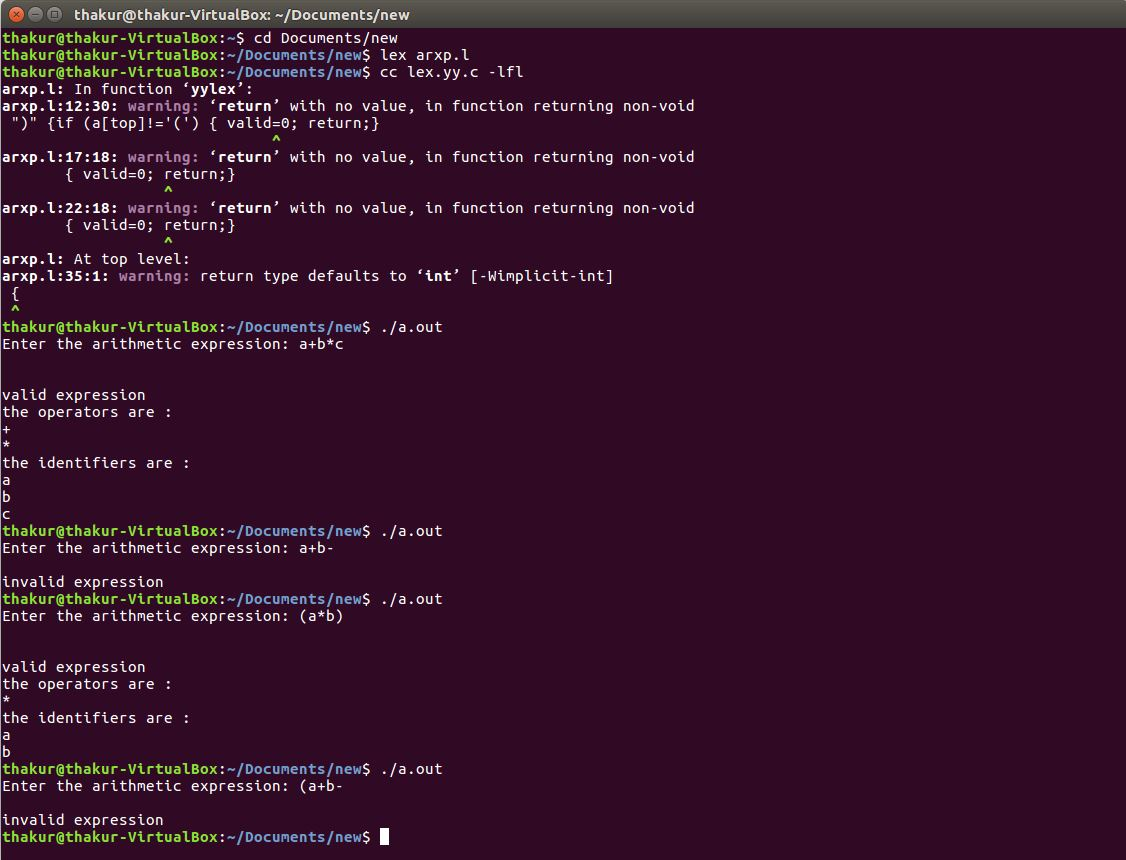# Lex program to recognize valid arithmetic expression and identify the identifiers and operators

• Last Updated : 03 Dec, 2019

Problem: Write a Lex program to recognize valid arithmetic expression and identify the identifiers and operators.

Explanation:
Flex (Fast lexical Analyzer Generator) is a tool/computer program for generating lexical analyzers (scanners or lexers) written by Vern Paxson in C around 1987. Lex reads an input stream specifying the lexical analyzer and outputs source code implementing the lexer in the C programming language. The function yylex() is the main flex function which runs the Rule Section.

Attention reader! Don’t stop learning now. Get hold of all the important CS Theory concepts for SDE interviews with the CS Theory Course at a student-friendly price and become industry ready.

Examples:

```Input: a+b*c
Output: valid expression
the operators are :
+
*
the identifiers  are :
a
b
c

Input: a+b-
Output: invalid expression

Input: (a*b)
Output: valid expression
the operators are :
*
the identifiers are :
a
b

Input: (a+b-
Output: invalid expression ```

Implementation:

 `/* Lex program to recognize valid arithmetic expression``        ``and identify the identifiers and operators */``%{ ``#include  ``#include  ``    ``int` `operators_count = 0, operands_count = 0, valid = 1, top = -1, l = 0, j = 0; ``    ``char` `operands, operators, stack; ``%} ``%%``"("` `{ ``    ``top++; ``    ``stack[top] = ``'('``; ``} ``"{"` `{``    ``top++; ``    ``stack[top] = ``'{'``; ``} ``"["` `{ ``    ``top++; ``    ``stack[top] = ``'['``; ``} ``")"` `{ ``    ``if` `(stack[top] != ``'('``) { ``        ``valid = 0;     ``    ``} ``    ``else` `if``(operands_count>0 && (operands_count-operators_count)!=1){``        ``valid=0;``    ``}``    ``else``{``        ``top--;``        ``operands_count=1;``        ``operators_count=0;``    ``} ``} ``"}"` `{ ``    ``if` `(stack[top] != ``'{'``) { ``        ``valid = 0;     ``    ``} ``    ``else` `if``(operands_count>0 && (operands_count-operators_count)!=1){``        ``valid=0;``    ``}``    ``else``{``        ``top--;``        ``operands_count=1;``        ``operators_count=0;``    ``} ``} ``"]"` `{ ``    ``if` `(stack[top] != ``'['``) { ``        ``valid = 0; ``    ``} ``    ``else` `if``(operands_count>0 && (operands_count-operators_count)!=1){``        ``valid=0;``    ``}``    ``else``{``        ``top--;``        ``operands_count=1;``        ``operators_count=0;``    ``} ``         ` `} ``"+"``|``"-"``|``"*"``|``"/"` `{ ``    ``operators_count++; ``    ``strcpy``(operators[l], yytext); ``    ``l++; ``} ``[0-9]+|[a-zA-Z][a-zA-Z0-9_]* { ``    ``operands_count++; ``    ``strcpy``(operands[j], yytext); ``    ``j++; ``} ``%% `` ` ` ` `int` `yywrap() ``{ ``    ``return` `1; ``} ``int` `main() ``{ ``    ``int` `k; ``    ``printf``(``"Enter the arithmetic expression: "``); ``    ``yylex(); `` ` `    ``if` `(valid == 1 && top == -1) { ``        ``printf``(``"\nValid Expression\n"``); ``    ``} ``    ``else``        ``printf``(``"\nInvalid Expression\n"``); `` ` `    ``return` `0;``} `

Output:My Personal Notes arrow_drop_up